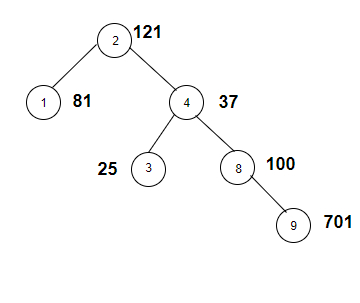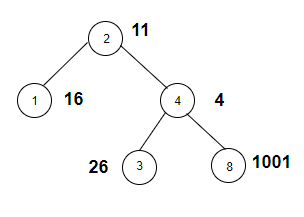# Count the nodes whose weight is a perfect square in C++

Given a binary tree with weights of its nodes. The goal is to find the number of nodes that have weights such that the number is a perfect square. If weight is 36 then it is 62 so this node will be counted.

For Example

## Input

The tree which will be created after inputting the values is given below −## Output

Count the nodes whose weight is a perfect square are: 4

## Explanation

we are given with the tree nodes and the weights associated with each node. Now we check whether the digits of nodes are perfect squares or not.

NodeWeightPerfect squareYes/no
212111*11yes
1819*9yes
437Prime numberno
3255*5yes
810010*10yes
9701Not possibleno

## Input

The tree which will be created after inputting the values is given below −## Output

Count the nodes whose weight is a perfect square are: 2

## Explanation

we are given with the tree nodes and the weights associated with each
node. Now we check whether the digits of nodes are perfect squares or not.

NodeWeightPerfect squareYes / No
211Not possibleno
1164*4yes
442*2yes
326Not possibleno
81001Not possibleno

Approach used in the below program is as follows

In this approach we will apply DFS on the graph of the tree to traverse it and check if the weight of the node is a perfect square. Take two vectors Node_Weight(100) and edge_graph for this purpose.

• Initialize Node_Weight[] with the weights of nodes.

• Create a tree using vector edge_graph.

• Take a global variable square and initialize it with 0.

• Function check(int check_it) takes an integer and returns true if check_it is a perfect square.

• Take total = sqrt(check_it)

• Now if(floor(total) != ceil(total)) returns true then total is not a perfect square, return false.

• Return true otherwise.

• Function perfect_square(int node, int root) takes a node and root node of a tree and returns the count of nodes in the given tree whose weight is a perfect square.

• If if(check(Node_Weight[node])) returns true, then increment square.

• Traverse tree in vector edge_graph[node] using for loop.

• Call perfect_square(it, node) for the next node in the vector.

• At the end of all functions we will have a square as the number of nodes with weights having value as a perfect square.

## Example

Live Demo

#include <bits/stdc++.h>
using namespace std;
vector<int> Node_Weight(100);
vector<int> edge_graph;
int square = 0;
bool check(int check_it){
double total = sqrt(check_it);
if(floor(total) != ceil(total)){
return false;
}
return true;
}
void perfect_square(int node, int root){
if(check(Node_Weight[node])){
square++;
}
for (int it : edge_graph[node]){
if(it == root){
continue;
}
perfect_square(it, node);
}
}
int main(){
//weight of the nodes
Node_Weight = 121;
Node_Weight = 81;
Node_Weight = 37;
Node_Weight = 25;
Node_Weight = 100;
Node_Weight = 701;
//create graph edge
edge_graph.push_back(1);
edge_graph.push_back(4);
edge_graph.push_back(3);
edge_graph.push_back(8);
edge_graph.push_back(9);
perfect_square(2, 2);
cout<<"Count the nodes whose weight is a perfect square are: "<<square;
return 0;
}

## Output

If we run the above code it will generate the following output −

Count the nodes whose weight is a perfect square are: 4